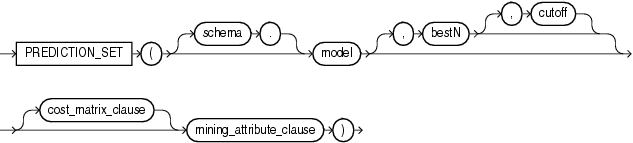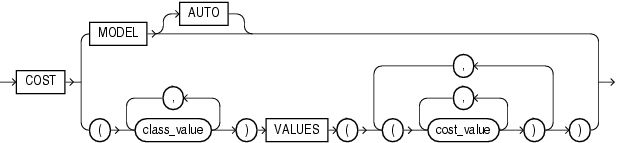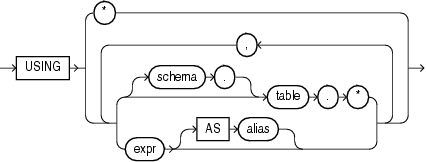# PREDICTION_SET

SyntaxDescription of the illustration prediction_set.gif

cost_matrix_clause::=Description of the illustration cost_matrix_clause.gif

mining_attribute_clause::=Description of the illustration mining_attribute_clause.gif

Purpose

This function is for use with classification models created using the `DBMS_DATA_MINING` package or with the Oracle Data Mining Java API. It is not valid with other types of models. It returns a varray of objects containing all classes in a multiclass classification scenario. The object fields are named `PREDICTION`, `PROBABILITY`, and `COST`. The datatype of the `PREDICTION` field depends on the target value type used during the build of the model. The other two fields are both Oracle `NUMBER`. The elements are returned in the order of best prediction to worst prediction.

• For `bestN`, specify a positive integer to restrict the returned target classes to the `N` having the highest probability, or lowest cost if cost matrix clause is specified. If multiple classes are tied in the Nth value, then the database still returns only `N` values. If you want to filter only by `cutoff`, specify `NULL` for this parameter.

• For `cutoff`, specify a `NUMBER` value to restrict the returned target classes to those with a probability greater than or equal to (or a cost less than or equal to if cost matrix clause is specified) to the specified cutoff value. You can filter solely by `cutoff` by specifying `NULL` for `bestN`.

When you specify values for both `bestN` and `cutoff`, you restrict the returned predictions to only those that are the `bestN` and have a probability (or cost when the `cost_matrix_clause` is specified) surpassing the threshold.

The `cost_matrix_clause` clause is relevant for all classification models. When you specify this clause, both `bestN` and `cutoff` are treated with respect to the prediction cost, not the prediction probability. The value of `bestN` restricts the result to the target classes having the `N` best (lowest) costs, and `cutoff` restricts the target classes to those with a cost less than or equal to the specified cutoff.

When you specify this clause, each object in the collection is a triplet of scalar values containing the prediction value (the datatype of which depends on the target value type used during model build), the prediction probability, and the prediction cost (both Oracle `NUMBER`).

If you omit this clause, then each object in the varray is a pair of scalars containing the prediction value and prediction probability. The datatypes returned are as described in the preceding paragraph.

• Specify `COST` `MODEL` to indicate that the scoring should be performed by taking into account the scoring cost matrix associated with the model. If no such cost matrix exists, then the database returns an error.

• Specify `COST` `MODEL` `AUTO` if the existence of a cost matrix is unknown. In this case:

• If the stored cost matrix exists, then the result is the same as with `COST` `MODEL`.

• If no stored cost matrix exists, then the result is almost the same as without the `cost_matrix_clause`, except the object in the collection is a triplet and the cost value is computed based on the unit cost matrix (0's on the diagonal and 1's everywhere else). This is equivalent to one minus probability for the given class. The cutoff parameter is ignored if no stored cost matrix exists.

• Use the `VALUES` clause (the bottom branch of the `cost_matrix_clause`) to specify an inline cost matrix. You can use an inline cost matrix regardless of whether the model has an associated scoring cost matrix.

The `mining_attribute_clause` behaves as described for the `PREDICTION` function. Refer to mining_attribute_clause.

Example

The following example lists, for ten customers, the likelihood and cost of using or rejecting an affinity card. This example has a binary target, but such a query is also useful in multiclass classification such as Low, Med, and High.

This example and the prerequisite data mining operations can be found in the demo file `\$ORACLE_HOME/rdbms/demo/dmdtdemo.sql`. General information on data mining demo files is available in Oracle Data Mining Administrator's Guide. The example is presented here to illustrate the syntactic use of the function.

```SELECT T.cust_id, S.prediction, S.probability, S.cost
FROM (SELECT cust_id,
PREDICTION_SET(dt_sh_clas_sample COST MODEL USING *) pset
FROM mining_data_apply_v
WHERE cust_id < 100011) T,
TABLE(T.pset) S
ORDER BY cust_id, S.prediction;

CUST_ID PREDICTION PROBABILITY  COST
---------- ---------- ----------- -----
100001          0      .96682   .27
100001          1      .03318   .97
100002          0      .74038  2.08
100002          1      .25962   .74
100003          0      .90909   .73
100003          1      .09091   .91
100004          0      .90909   .73
100004          1      .09091   .91
100005          0      .27236  5.82
100005          1      .72764   .27
100006          0     1.00000   .00
100006          1      .00000  1.00
100007          0      .90909   .73
100007          1      .09091   .91
100008          0      .90909   .73
100008          1      .09091   .91
100009          0      .27236  5.82
100009          1      .72764   .27
100010          0      .80808  1.54
100010          1      .19192   .81

20 rows selected.
```